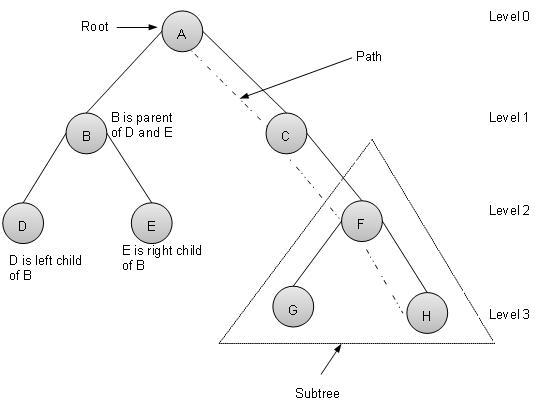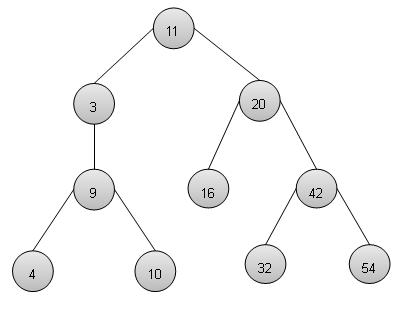# DSA using C - Tree

## Overview

Tree represents nodes connected by edges. We'll going to discuss binary tree or binary search tree specifically.

Binary Tree is a special datastructure used for data storage purposes. A binary tree has a special condition that each node can have two children at maximum. A binary tree have benefits of both an ordered array and a linked list as search is as quick as in sorted array and insertion or deletion operation are as fast as in linked list.## Terms

Following are important terms with respect to tree.

• Path − Path refers to sequence of nodes along the edges of a tree.

• Root − Node at the top of the tree is called root. There is only one root per tree and one path from root node to any node.

• Parent − Any node except root node has one edge upward to a node called parent.

• Child − Node below a given node connected by its edge downward is called its child node.

• Leaf − Node which does not have any child node is called leaf node.

• Subtree − Subtree represents descendents of a node.

• Visiting − Visiting refers to checking value of a node when control is on the node.

• Traversing − Traversing means passing through nodes in a specific order.

• Levels − Level of a node represents the generation of a node. If root node is at level 0, then its next child node is at level 1, its grandchild is at level 2 and so on.

• keys − Key represents a value of a node based on which a search operation is to be carried out for a node.

Binary Search tree exibits a special behaviour. A node's left child must have value less than its parent's value and node's right child must have value greater than it's parent value.

## Binary Search Tree RepresentationWe're going to implement tree using node object and connecting them through references.

## Basic Operations

Following are basic primary operations of a tree which are following.

• Search − search an element in a tree.

• Insert − insert an element in a tree.

• Preorder Traversal − traverse a tree in a preorder manner.

• Inorder Traversal − traverse a tree in an inorder manner.

• Postorder Traversal − traverse a tree in a postorder manner.

## Node

Define a node having some data, references to its left and right child nodes.

```struct node {
int data;
struct node *leftChild;
struct node *rightChild;
};
```

## Search Operation

Whenever an element is to be search. Start search from root node then if data is less than key value, search element in left subtree otherwise search element in right subtree. Follow the same algorithm for each node.

```struct node* search(int data){
struct node *current = root;
printf("Visiting elements: ");
while(current->data != data){
if(current != NULL)
printf("%d ",current->data);
//go to left tree
if(current->data > data){
current = current->leftChild;
}//else go to right tree
else{
current = current->rightChild;
}
if(current == NULL){
return NULL;
}
}
return current;
}
```

## Insert Operation

Whenever an element is to be inserted. First locate its proper location. Start search from root node then if data is less than key value, search empty location in left subtree and insert the data. Otherwise search empty location in right subtree and insert the data.

```void insert(int data){
struct node *tempNode = (struct node*) malloc(sizeof(struct node));
struct node *current;
struct node *parent;

tempNode->data = data;
tempNode->leftChild = NULL;
tempNode->rightChild = NULL;

//if tree is empty
if(root == NULL){
root = tempNode;
} else {
current = root;
parent = NULL;

while(1){
parent = current;
//go to left of the tree
if(data < parent->data){
current = current->leftChild;
//insert to the left
if(current == NULL){
parent->leftChild = tempNode;
return;
}
}//go to right of the tree
else{
current = current->rightChild;
//insert to the right
if(current == NULL){
parent->rightChild = tempNode;
return;
}
}
}
}
}
```

## Preorder Traversal

It is a simple three step process.

• visit root node

• traverse left subtree

• traverse right subtree

```void preOrder(struct node* root){
if(root!=NULL){
printf("%d ",root->data);
preOrder(root->leftChild);
preOrder(root->rightChild);
}
}
```

## Inorder Traversal

It is a simple three step process.

• traverse left subtree

• visit root node

• traverse right subtree

```void inOrder(struct node* root){
if(root!=NULL){
inOrder(root->leftChild);
printf("%d ",root->data);
inOrder(root->rightChild);
}
}
```

## Postorder Traversal

It is a simple three step process.

• traverse left subtree

• traverse right subtree

• visit root node

```void postOrder(struct node* root){
if(root!=NULL){
postOrder(root->leftChild);
postOrder(root->rightChild);
printf("%d ",root->data);
}
}
```

## Example

```#include <stdio.h>
#include <string.h>
#include <stdlib.h>
#include <stdbool.h>

struct node {
int data;
struct node *leftChild;
struct node *rightChild;
};
struct node *root = NULL;
void insert(int data){
struct node *tempNode = (struct node*) malloc(sizeof(struct node));
struct node *current;
struct node *parent;

tempNode->data = data;
tempNode->leftChild = NULL;
tempNode->rightChild = NULL;

//if tree is empty
if(root == NULL){
root = tempNode;
} else {
current = root;
parent = NULL;
while(1){
parent = current;
//go to left of the tree
if(data < parent->data){
current = current->leftChild;
//insert to the left
if(current == NULL){
parent->leftChild = tempNode;
return;
}
}//go to right of the tree
else{
current = current->rightChild;
//insert to the right
if(current == NULL){
parent->rightChild = tempNode;
return;
}
}
}
}
}
struct node* search(int data){
struct node *current = root;
printf("Visiting elements: ");
while(current->data != data){
if(current != NULL)
printf("%d ",current->data);
//go to left tree
if(current->data > data){
current = current->leftChild;
}//else go to right tree
else{
current = current->rightChild;
}
if(current == NULL){
return NULL;
}
}
return current;
}
void preOrder(struct node* root){
if(root!=NULL){
printf("%d ",root->data);
preOrder(root->leftChild);
preOrder(root->rightChild);
}
}
void inOrder(struct node* root){
if(root!=NULL){
inOrder(root->leftChild);
printf("%d ",root->data);
inOrder(root->rightChild);
}
}
void postOrder(struct node* root){
if(root!=NULL){
postOrder(root->leftChild);
postOrder(root->rightChild);
printf("%d ",root->data);
}
}
void traverse(int traversalType){
switch(traversalType){
case 1:
printf("\nPreorder traversal: ");
preOrder(root);
break;
case 2:
printf("\nInorder traversal: ");
inOrder(root);
break;
case 3:
printf("\nPostorder traversal: ");
postOrder(root);
break;
}
}

int main()
{
/*                     11               //Level 0
*/
insert(11);
/*                     11               //Level 0
*                      |
*                      |---20           //Level 1
*/
insert(20);
/*                     11               //Level 0
*                      |
*                  3---|---20           //Level 1
*/
insert(3);
/*                     11               //Level 0
*                      |
*                  3---|---20           //Level 1
*                           |
*                           |--42       //Level 2
*/
insert(42);
/*                     11               //Level 0
*                      |
*                  3---|---20           //Level 1
*                           |
*                           |--42       //Level 2
*                               |
*                               |--54   //Level 3
*/
insert(54);
/*                     11               //Level 0
*                      |
*                  3---|---20           //Level 1
*                           |
*                       16--|--42       //Level 2
*                               |
*                               |--54   //Level 3
*/
insert(16);
/*                     11               //Level 0
*                      |
*                  3---|---20           //Level 1
*                           |
*                       16--|--42       //Level 2
*                               |
*                           32--|--54   //Level 3
*/
insert(32);
/*                     11               //Level 0
*                      |
*                  3---|---20           //Level 1
*                  |        |
*                  |--9 16--|--42       //Level 2
*                               |
*                           32--|--54   //Level 3
*/
insert(9);
/*                     11               //Level 0
*                      |
*                  3---|---20           //Level 1
*                  |        |
*                  |--9 16--|--42       //Level 2
*                     |         |
*                  4--|     32--|--54   //Level 3
*/
insert(4);
/*                     11               //Level 0
*                      |
*                  3---|---20           //Level 1
*                  |        |
*                  |--9 16--|--42       //Level 2
*                     |         |
*                  4--|--10 32--|--54   //Level 3
*/
insert(10);

struct node * temp = search(32);
if(temp!=NULL){
printf("Element found.\n");
printf("( %d )",temp->data);
printf("\n");
} else {
}

struct node *node1 = search(2);
if(node1!=NULL){
printf("Element found.\n");
printf("( %d )",node1->data);
printf("\n");
} else {
}

//pre-order traversal
//root, left ,right
traverse(1);
//in-order traversal
//left, root ,right
traverse(2);
//post order traversal
//left, right, root
traverse(3);
}
```

## Output

If we compile and run the above program then it would produce following output −

```Visiting elements: 11 20 42 Element found.(32)